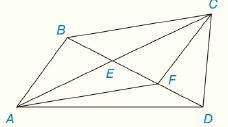Chapter 4.CR, Problem 27CR### Elementary Geometry for College St...

6th Edition
Daniel C. Alexander + 1 other
ISBN: 9781285195698

#### Solutions

Chapter
Section### Elementary Geometry for College St...

6th Edition
Daniel C. Alexander + 1 other
ISBN: 9781285195698
Textbook Problem
1 views

# Review Exercises Given: D E is a median of ∆ A D C B E - ≅ F D - E F - ≅ F D - Prove: A B C D is a ▱To determine

To Prove:

The provided quadrilateral ABCF is a parallelogram.

Explanation

Proof:

Definition:

A median of a triangle is a line segment joining a vertex to the midpoint of the opposite side, thus bisecting that side. Every triangle has exactly three medians, one from each vertex, and they all intersect each other at the triangle’s centroid.

Theorem on parallelogram:

If the diagonals of a quadrilateral bisect each other, then it is a parallelogram.

Description:

It is given that DE is a median of ADC with BE-FD- and EF-FD-.

BE-FD-EF-. That is, BE-EF-

### Still sussing out bartleby?

Check out a sample textbook solution.

See a sample solution

#### The Solution to Your Study Problems

Bartleby provides explanations to thousands of textbook problems written by our experts, many with advanced degrees!

Get Started

#### Evaluate: 182536+47

Elementary Technical Mathematics

#### Solve the equations in Exercises 126. (x+1)2(2x+3)(x+1)(2x+3)2=0

Finite Mathematics and Applied Calculus (MindTap Course List)

#### Define (a) a polynomial function and (b) a rational function. Give an example of each.

Applied Calculus for the Managerial, Life, and Social Sciences: A Brief Approach

#### Evaluate the integrals in Problems 7-18. 13.

Mathematical Applications for the Management, Life, and Social Sciences

#### True or False: converges mean exists.

Study Guide for Stewart's Multivariable Calculus, 8th